﻿ 一种改进的卷积神经网络行人识别方法
«上一篇文章快速检索 高级检索

 应用科技2019, Vol. 46Issue (3): 51-57  DOI: 10.11991/yykj.2018090140

### 引用本文CHEN Cong, YANG Zhong, SONG Jiarong, et al. An improved pedestrian detection method based on convolutional neural network[J]. Applied Science and Technology, 2019, 46(3), 51-57. DOI: 10.11991/yykj.201809014.### 文章历史

An improved pedestrian detection method based on convolutional neural network
CHEN Cong , YANG Zhong, SONG Jiarong , HAN Jiaming
College of Automation Engineering, Nanjing University of Aeronautics and Astronautics, Nanjing 211106, China
Abstract: To solve the problem of poor positioning accuracy and low real-time performance in current pedestrian detection algorithms, a real-time pedestrian detection method is proposed by applying the You Only Look Once (YOLO) algorithm of target detection. Based on the Tiny−YOLO network, by changing the size of input of the network model, it helps the network gain better expression of pedestrian characteristics. Combined with the pedestrian size characteristics in the image, the cluster analysis method is used to calculate the initial candidate boxes in dataset, and the size and number of anchor boxes suitable for pedestrian detection are selected. Convolution layers are added in order to redesign the feature extraction and target detection networks. Train the network on a mixed dataset to improve the generalization of the model. The experimental results show that the method has lower miss rate, better positioning accuracy and detection effect when it comes to the situation of different sized pedestrians and partial occlusion, and the detection speed can meet the real-time requirements.
Keywords: pedestrian detection    convolutional neural network(CNN)    deep learning    YOLO    feature extraction    cluster analysis    multi-scale feature    pedestrian dataset

1 Tiny−YOLO原理 1.1 Tiny−YOLO网络结构

Tiny−YOLO主干网络拥有7个3×3的卷积(convolution)层，6个池化(maxpooling)层，前5为个步长为2的池化层，最后一个为步长为1的池化层。模型输入为416 pix×416 pix，在经过5个步长为2的池化层后，最终特征图大小为13×13。网络结构如图1所示。

1)在基础网络之后添加一些卷积层进行预测，此时特征图大小为13 pix×13 pix。尺度1)对大尺寸目标有较好的预测能力。

2)从尺度1)中的倒数第2层的卷积层进行2倍上采样，上采样可以帮助网络学习细粒度特征，与最后一个尺寸为26 pix×26 pix的特征图相加，再次通过多个卷积后输出预测信息，特征图大小为26 pix×26 pix。尺度2)使用了较大的特征图，对较小尺寸的目标有更好的预测能力。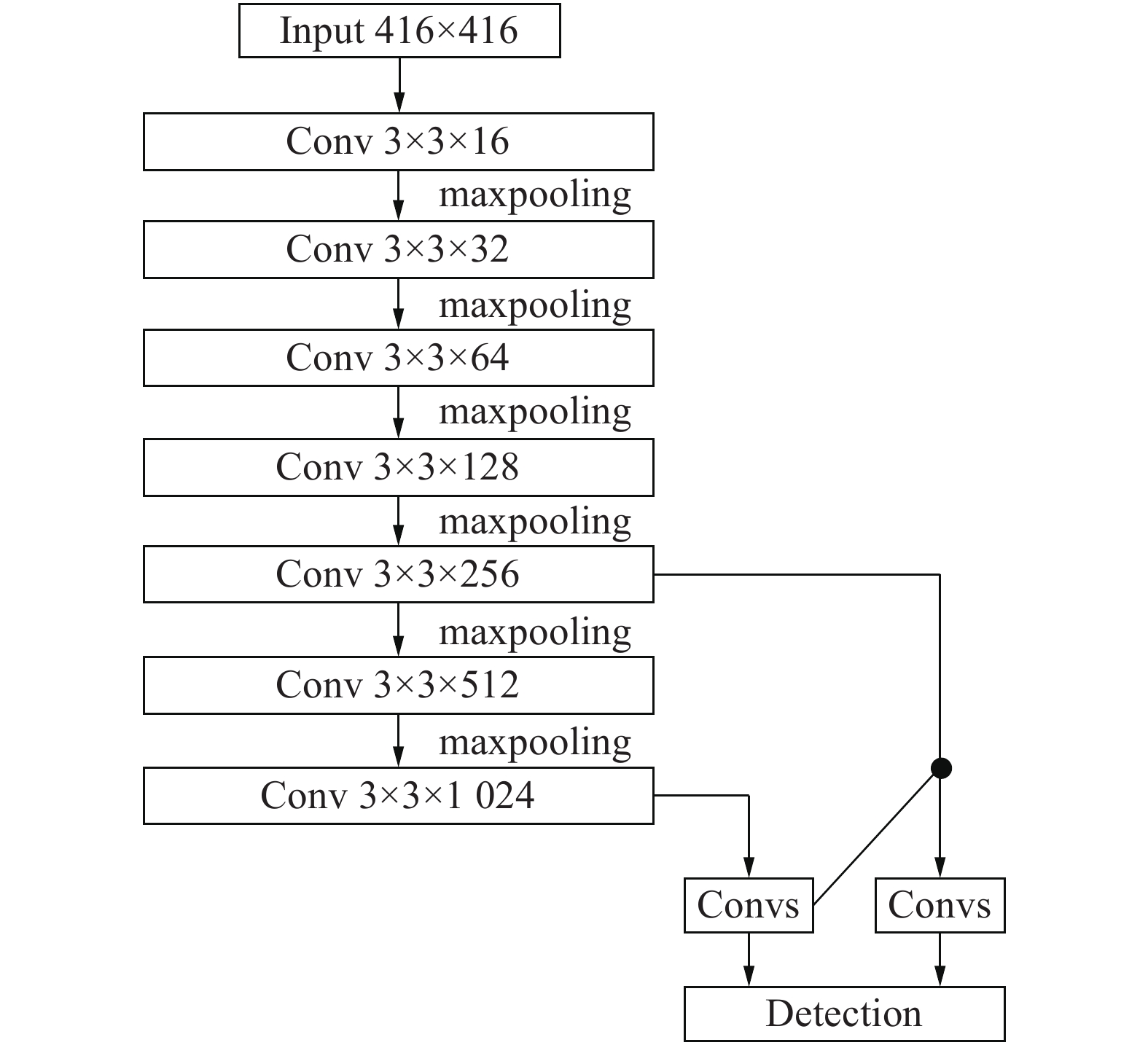Download: 图 1 Tiny-YOLO网络结构
1.2 损失函数

 $E = \sum\limits_{i = 0}^{{s^2}} {{E_{{\rm{cood}}}} + {E_{{\rm{IOU}}}} + {E_{{\rm{class}}}}}$

 \begin{aligned} & {\lambda _{{\mathop{\rm coord}\nolimits} }}\sum\limits_{i = 0}^{{S^2}} {\sum\limits_{j = 0}^B {\prod {\sum {I_{ij}^{{\rm{person}}}[{{({x_i} - {{\hat x}_i})}^2} + {{({y_i} - {{\hat y}_i})}^2}]} } } } {\rm{ + }}\\ & \quad \quad {\lambda _{{\rm{coord}}}}\sum\limits_{i = 0}^{{S^2}} {\sum\limits_{j = 0}^B {\prod {\sum {I_{ij}^{{\rm{person}}}[{{(\sqrt {{w_i}} - \sqrt {{{\hat w}_i}} )}^2} + } } } } \\ & \quad \quad {(\sqrt {{h_i}} - \sqrt {{{\hat h}_i}} )^2}] + \sum\limits_{i = 0}^{{S^2}} {\sum\limits_{j = 0}^B {\prod {\sum {I_{ij}^{{\rm{person}}}{{({c_i} - {{\hat c}_i})}^2}} } } } {\rm{ + }}\\ & \quad \quad {\lambda _{{\rm{noperson}}}}\sum\limits_{i = 0}^{{S^2}} {\sum\limits_{j = 0}^B {\prod {\sum {I_{ij}^{{\rm{noperson}}}{{({c_i} - {{\hat c}_i})}^2}} } } } + \\ & \quad \quad \sum\limits_{i = 0}^{{S^2}} {{{\prod {\sum {I_i^{{\rm{person}}}\sum\limits_{c \in {\rm{classes}}} {({p_i}(c) - {{\hat p}_i}(c))} } } }^2}} \end{aligned}

1.3 激活函数

 $f(x) = \max (x,0.1x)$
1.4 边界框预测

Tiny-YOLO使用由维度聚类得到anchor box作为先验框来预测边界框(bounding box)：

 \left\{ \begin{aligned} & {b_x} = \sigma ({t_x}) + {c_x}\\ & {b_y} = \sigma ({t_y}) + {c_y}\\ & {b_w} = {p_w}{{\rm{e}}^{{t_w}}}\\ & {b_h} = {p_h}{{\rm{e}}^{{t_h}}} \end{aligned} \right.

2 改进的Ped−YOLO网络

Tiny−YOLO网络作为针对多类别目标检测的一种简化网络，已经取得了较好的检测效果和实时性，但并不完全适合行人检测任务，因此，需要针对具体问题进行相应的改进。

1)改变训练时输入图像的分辨率；

2)对行人检测数据集的目标框进行聚类，确定anchor参数；

3)改进网络结构，使用更深的卷积神经网络对行人特征进行提取。

2.1 优化输入图像分辨率

2.2 K-means聚类分析

YOLO系列算法引入了Faster R−CNN中的anchor boxes的思想，在数据集上进行聚类确定anchor boxes。YOLOv2的初始候选框anchor参数针对PASCAL VOC数据集进行聚类确定，聚类结果为5，因此其anchor数目为5；YOLOv3初始候选框anchor参数针对COCO数据集聚类确定，聚类结果为9，因此其anchor的数目为9，分属3个不同预测尺度；Tiny-YOLO的anchor参数同样针对COCO数据集聚类确定，聚类结果为6，因此其anchor的个数为6，分属2个不同预测尺度。由于PASCAL VOC数据集与COCO数据集中类别较多，得到的anchor参数具有普适性，但是并不适用于行人检测。在行人检测中，行人的长宽比不会因行人的背景、姿态等发生大的改变，往往是一个相对固定的比值，因此需要对数据集进行聚类分析，重新选择最优的anchor个数和宽高维度。文中采用K-means聚类方法获得先验框的尺寸，得到anchor的个数和宽高维度。

 $d({\rm{box,centroid}}) = 1 - {\rm{IOU}}({\rm{box,centroid}})$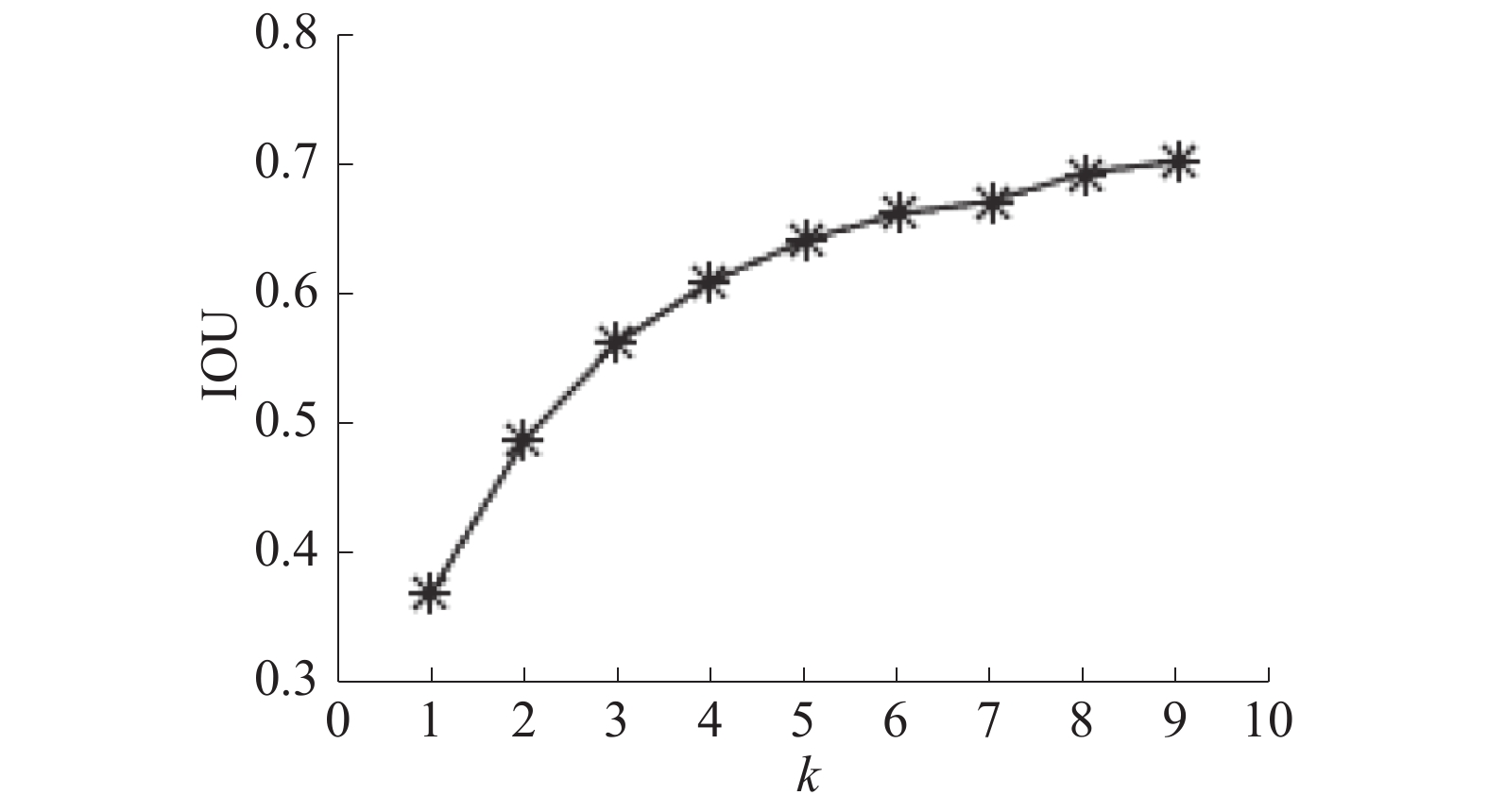Download: 图 2 IOU−k折线图

2.3 网络结构改进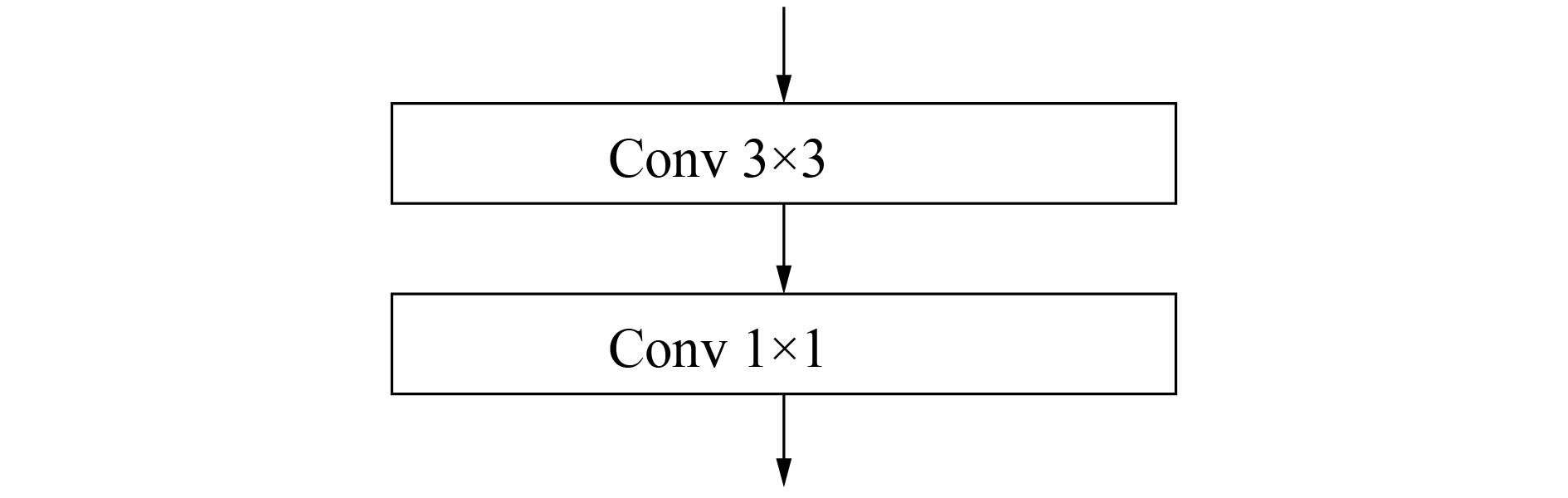Download: 图 3 Ped-YOLO中增加的卷积层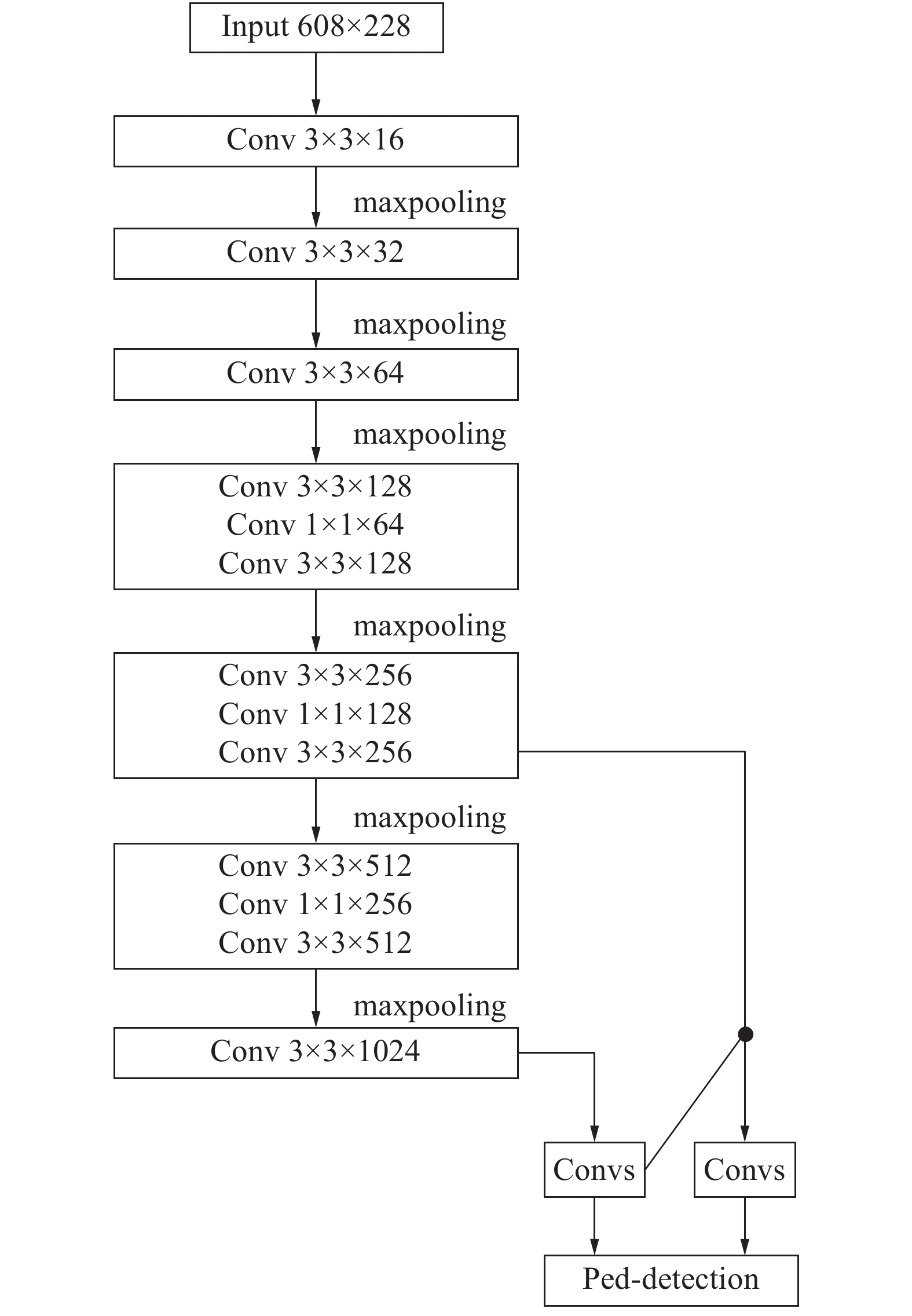Download: 图 4 Ped-YOLO网络结构
3 实验结果及分析 3.1 训练及测试数据集

3.2 实验平台表 1 实验平台软硬件配置
3.3 模型搭建与训练

3.4 实验结果与分析 3.4.1 混合数据集测试表 2 混合数据集测试结果
3.4.2 平均交并比测试表 3 平均交并比测试结果
3.4.3 精度测试表 4 精度测试结果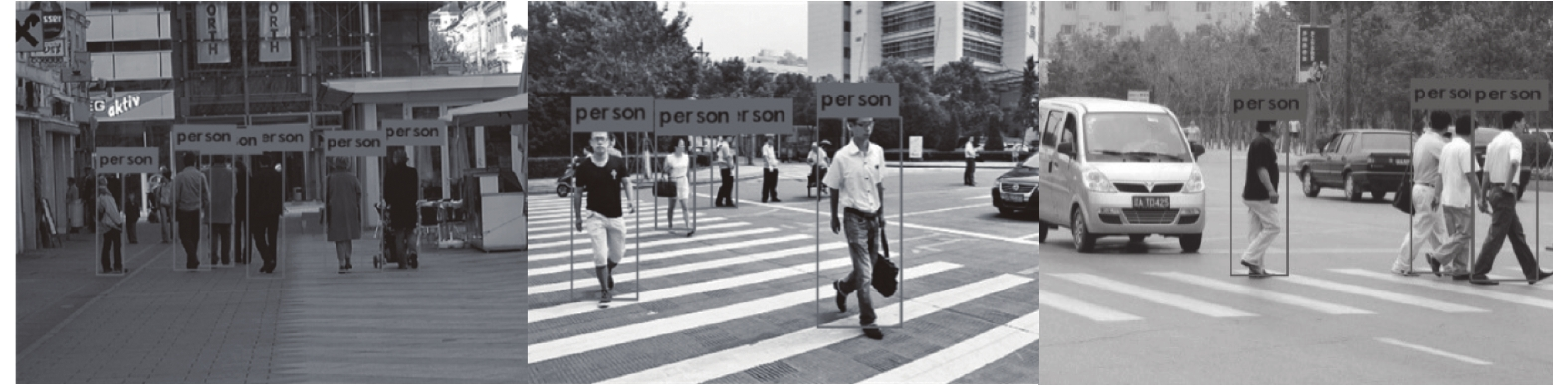Download: 图 5 Tiny−YOLO检测结果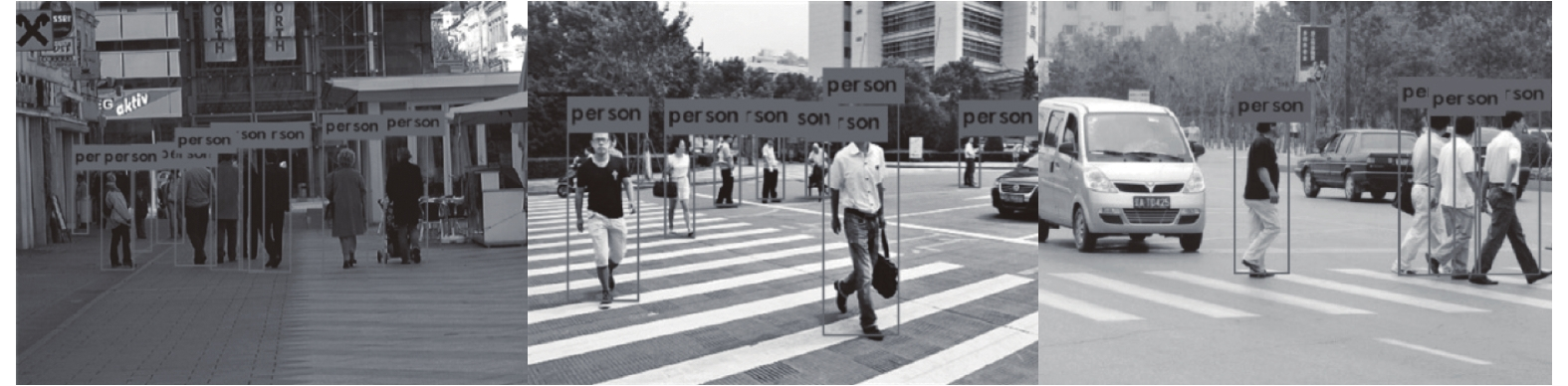Download: 图 6 Ped−YOLO检测结果

4 结论

1)与传统算法和Tiny−YOLO相比，Ped−YOLO网络具有更低的漏检率，Ped−YOLO网络在保持了较高的检测速度的同时，对不同尺寸、存在互相遮挡的行人有更好的检测效果。

2)本文提出的网络仍然存在一些问题，如训练受限于PASCAL VOC与INRIA数据集，行人训练样本偏少，对小目标行人检测效果偏差，行人被较大范围遮挡时，模型的检测效果也不强。因此，可以通过融合多类行人检测数据集进行网络训练，改善网络对不同尺寸、不同环境下行人的检测效果，增强模型的泛化能力。

3)在行人检测中，由于光照、遮挡等问题的存在，漏检率和误检率仍然达不到理想的水平。因此，需要提取更多的信息提升检测效果，如光流信息、语义信息等，提高特征表达能力，这也将成为行人检测的重要发展方向。

  SABZMEYDANI P, MORI G. Detecting pedestrians by learning shapelet features[C]//Proceedings of 2007 IEEE Conference on Computer Vision and Pattern Recognition. Minneapolis, MN, USA: IEEE, 2007: 1−8. (0)  WANG Xiaoyu, HAN T X, YAN Shuicheng. An HOG-LBP human detector with partial occlusion handling[C]//Proceedings of the 12th International Conference on Computer Vision. Kyoto: IEEE Press, 2009: 32−39. (0)  高宗, 李少波, 陈济楠, 等. 基于YOLO网络的行人检测方法[J]. 计算机工程, 2018, 44(5): 215-219. (0)  GIRSHICK R, DONAHUE J, DARRELL T, et al. Rich feature hierarchies for accurate object detection and semantic segmentation[C]//Proceedings of 2014 IEEE Conference on Computer Vision and Pattern Recognition. Columbus, OH, USA: IEEE, 2014. (0)  REN Shaoqing, HE Kaiming, GIRSHICK R, et al. Faster R-CNN: towards real-time object detection with region proposal networks[C]//Proceedings of the 28th International Conference on Neural Information Processing Systems. Montreal, Canada: ACM, 2015: 91−99. (0)  LIU Wei, ANGUELOV D, ERHAN D, et al. SSD: single shot multibox detector[C]//Proceedings of the 14th European Conference on Computer Vision. Amsterdam: Springer, 2016: 21−37. (0)  REDMON J, DIVVALA S, GIRSHICK R, et al. You only look once: unified, real-time object detection[C]// Proceedings of 2016 IEEE Conference on Computer Vision and Pattern Recognition. Las Vegas, NV, USA: IEEE, 2016: 779−788. (0)  REDMON J, FARHADI A. YOLO9000: better, faster, stronger[C]//Proceedings of 2017 IEEE Conference on Computer Vision and Pattern Recognition. Honolulu, HI, USA: IEEE, 2017: 6517−6525. (0)  REDMON J, FARHADI A. YOLOv3: an incremental improvement[J]. arXiv: 1804.02767, 2018 . (0)  REDMON J. Darknet: open source neural networks in C[DB/OL]. [2017-10-25]. http://pjreddie.com/darknet/. (0)  刘辉, 彭力, 闻继伟. 基于改进全卷积网络的多尺度感知行人检测算法[J]. 激光与光电子学进展, 2018, 55(9): 91504. (0)  冯帅, 张龙, 贺小慧. 基于Jetson TK1和深度卷积神经网络的行人检测[J]. 信息技术, 2017(10): 62-64. DOI:10.3969/j.issn.1674-2117.2017.10.025 (0)  MAO Jiayuan, XIAO Tete, JIANG Yuning, et al. What can help pedestrian detection?[C]//Proceedings of 2017 IEEE Conference on Computer Vision and Pattern Recognition. Honolulu, HI, USA: IEEE, 2017: 6034-6043. (0)  郝旭政, 柴争义. 一种改进的深度残差网络行人检测方法[J/OL]. 计算机应用研究, 2019, 36(6). [2018-05-22]. (2018-03-16) http://www.arocmag.com/article/02-2019-06-057.html. DOI: 10.3969/j.issn.1001-3695.2017.12.0836. (0)  DAI Jifeng, LI Yi, HE Kaiming, et al. R-FCN: object detection via region-based fully convolutional networks[C]//Proceedings of the 30th International Conference on Neural Information Processing Systems. Barcelona, Spain: ACM, 2016: 379−387. (0)  HE Kaiming, ZHANG Xiangyu, REN Shaoqing, et al. Deep residual learning for image recognition[C]// Proceedings of 2016 IEEE Conference on Computer Vision and Pattern Recognition. Las Vegas, NV, USA: IEEE, 2016: 770−778. (0)  HUANG Gao, LIU Zhuang, VAN DER MAATEN L, et al. Densely connected convolutional networks[C]// Proceedings of 2017 IEEE Conference on Computer Vision and Pattern Recognition. Honolulu, HI, USA: IEEE, 2017: 2261-2269. (0)  刘建国, 罗杰, 王帅帅, 等. 基于YOLOv2的行人检测方法研究[J]. 数字制造科学, 2018, 16(1): 50-54. (0)  DOLLAR P, WOJEK C, SCHIELE B, et al. Pedestrian detection: an evaluation of the state of the art[J]. IEEE transactions on pattern analysis and machine intelligence, 2012, 34(4): 743-761. DOI:10.1109/TPAMI.2011.155 (0)  DALAL N, TRIGGS B. Histograms of oriented gradients for human detection[C]//Proceedings of 2015 IEEE Computer Society Conference on Computer Vision and Pattern Recognition. San Diego, CA, USA: IEEE Computer Society, 2005: 886−893. (0)  FELZENSZWALB P F, GIRSHICK R B, MCALLESTER D, et al. Object detection with discriminatively trained part-based models[J]. IEEE transactions on pattern analysis and machine intelligence, 2010, 32(9): 1627-1645. DOI:10.1109/TPAMI.2009.167 (0)